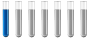## BALANCE CHEMICAL EQUATIONS

Chemistry and homework help forum.

Organic Chemistry, Analytical Chemistry, Biochemistry, Physical Chemistry, Computational Chemistry, Theoretical Chemistry, High School Chemistry, Colledge Chemistry and University Chemistry Forum.

Share your chemistry ideas, discuss chemical problems, ask for help with scientific chemistry questions, inspire others by your chemistry vision!

Please feel free to start a scientific chemistry discussion here!

Discuss chemistry homework problems with experts!

Ask for help with chemical questions and help others with your chemistry knowledge!

Moderators: expert, ChenBeier, Xen

bm9000
NewbiePosts: 3
Joined: Mon May 03, 2021 2:33 pm

### BALANCE CHEMICAL EQUATIONS

Does anybody have time to assist me balancing the following chemical equations ? I have started already with some. I do believe some equations cannot be solved. I'll appreciate any assistance.

NH3 + H3PO4 =
CO(NH2)2 + H3PO4 =
HNO3 + H3PO4 =
NH4OH + H3PO4 =
NH3 + KOH =
CO(NH2)2 + KOH =
HNO3 + KOH =
NH4OH + KOH =
NH3 + KCl =
CO(NH2)2 + KCl =
HNO3 + KCl =
NH4OH + KCl =
NH3 + K2CO3 =
CO(NH2)2 + K2CO3 =
HNO3 + K2CO3 =
NH4OH + K2CO3 =
NH3 + H3PO4 + KOH =
CO(NH2)2 + H3PO4 + KOH =
HNO3 + H3PO4 + KOH =
NH4OH + H3PO4 + KOH =
NH3 + H3PO4 + KCl =
CO(NH2)2 + H3PO4 + KCl =
HNO3 + H3PO4 + KCl =
NH4OH + H3PO4 + KCl =
NH3 + H3PO4 + K2CO3 =
CO(NH2)2 + H3PO4 + K2CO3 =
HNO3 + H3PO4 + K2CO3 =
NH4OH + H3PO4 + K2CO3 =

Regards,
BM9000
ChenBeier
Distinguished MemberPosts: 773
Joined: Wed Sep 27, 2017 7:25 am
Location: Berlin, Germany

### Re: BALANCE CHEMICAL EQUATIONS

What do you expect now. Better is you write your ideas, then we can discuss.
bm9000
NewbiePosts: 3
Joined: Mon May 03, 2021 2:33 pm

### Re: BALANCE CHEMICAL EQUATIONS

As mentioned, I've started balancing some of the equations. I am struggling though when there are 3 reactants. I really want to look for compatibility of mixing.

The background - I've looked at the nutrient constituents on some complex NPK fertilisers. With the exception of Urea (also somewhat puzzling, because the final product contains H2O too), the rest do not correspond with theoretical calculations.

Want to use these balanced equations (along with theoretical constituent determination), to ask a fertiliser supplier or two why the difference.

Regards,

BM9000
ChenBeier
Distinguished MemberPosts: 773
Joined: Wed Sep 27, 2017 7:25 am
Location: Berlin, Germany

### Re: BALANCE CHEMICAL EQUATIONS

Acid + Base gives salt + water.
So it depends how much from each is added what happend.
Like KOH + H3PO4 you can have 3 different results. KH2PO4, K2HPO4 and K3PO4.
Ammonia gives Ammonium NH4+
Urea decompose with acids to ammonia and carbondioxide.
Salt1 + acid1 gives Salt2 + acid2, in solution you have only ions
So will KCl + HNO3 give K+, H+, Cl- and NO3-
This would also happen if you mix KNO3 and HCl.
Mixture of two acids give only mixture nothing more. Adding base give mixture of the salts.
Pottasium carbonate will decompose with acids to carbondioxide.
bm9000
NewbiePosts: 3
Joined: Mon May 03, 2021 2:33 pm

### Re: BALANCE CHEMICAL EQUATIONS

Thanks SO much for time taken to respond. Appreciated.

Do you by any chance know of an online program / tool, in which 2 or more reactants can be input, with (a) predictive product(s) ?

Regards,

BM9000